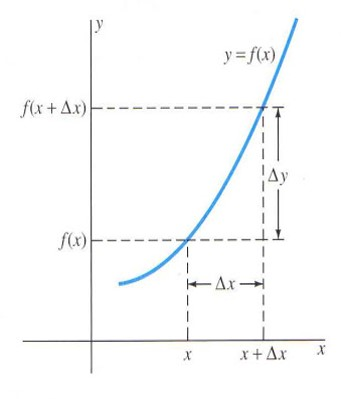# Points in a Plane

WELCOME STUDENTS!
TOPIC: INCREMENTS IN X & Y﻿

## Lets have a review about plotting points in Cartesian plane

When a particle moves from one point to another in the Cartesian Plane, there are changes in its coordinates. These net changes, called the increments of the coordinates, are determined by subtracting the coordinates of the starting point (initial point) from corresponding coordinates of the point where the particle stops (final point).In general, when a particle moves from  to the increment in x is and the increment in y is where ∆x (read as “delta x”) stands for the increment in x and ∆y (read as “delta y”) stands for the increment in y.

## Try to answer this

If the initial point is S(3, -2) and the final point is T(-1, 5) What is x?

Tick all that apply

If the initial point is S(3, -2) and the final point is T(-1, 5) What is y?

Tick all that apply

Find the increments of x and y if the initial point is A and the final point is B Show your solution. ﻿## Determine the increments in x and y as a particle moves from Point 1 to Point 2

1. (1, 5) to (4, 7)
2. (-2, 1) to (3, 1)

God Bless and Mabuhay!